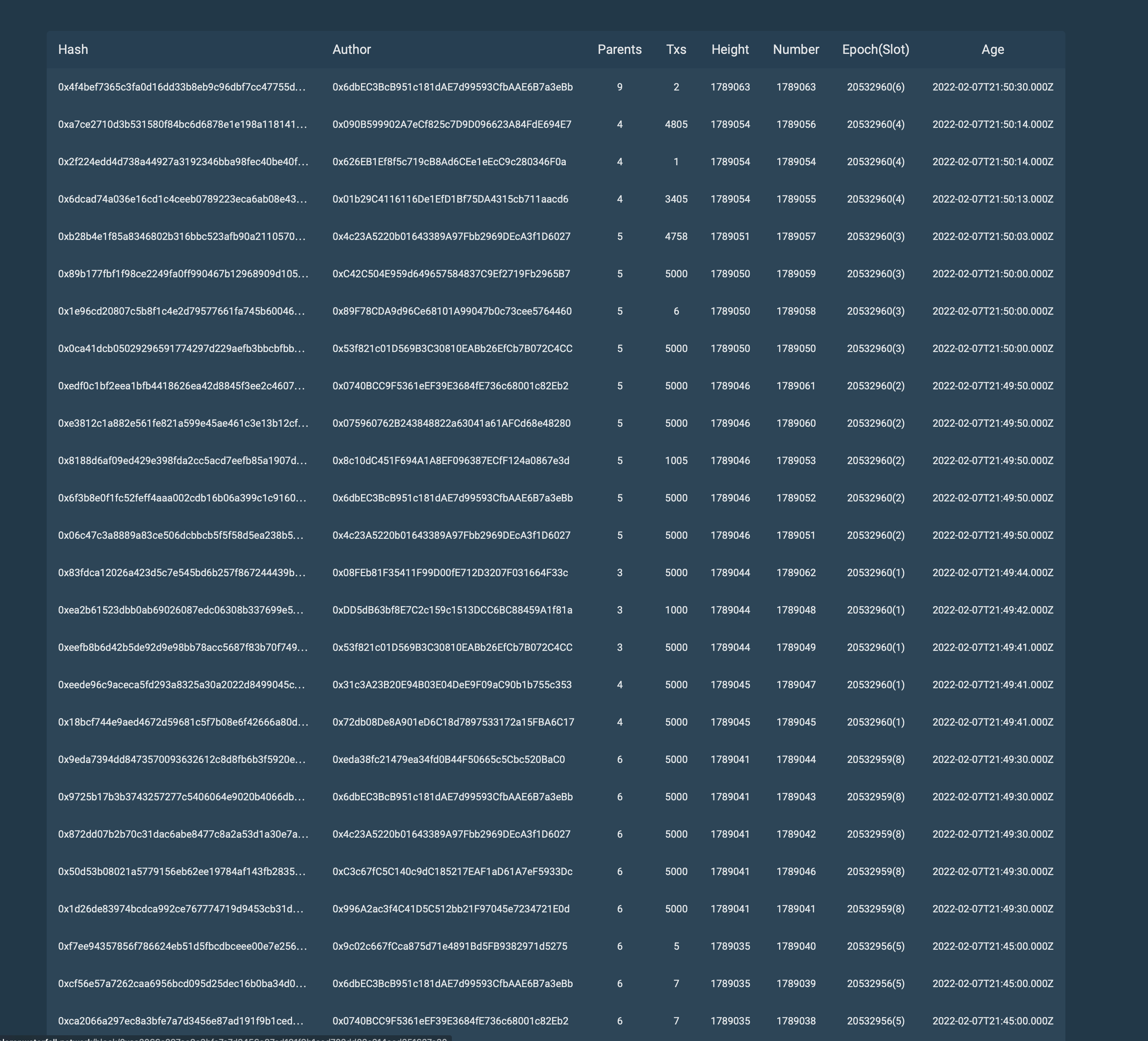# Testnet 2 Test 4

For the test, we used Amazon EC2 T3 Instances. With the following server characteristics:

• Name: `t3a.small`
• vCPUs: `2`
• Memory (GiB): `2.0`
• Baseline Performance/vCPU: `20%`
• CPU Credits earned/hr: `24`
• Network burst bandwidth (Gbps): `5`
• EBS burst bandwidth (Mbps): `Up to 2,085`

Algorithm of actions during testing:

1. Stop creating blocks in the tested network.
2. Generates ~80,000 transactions.
3. Start 64 nodes.
4. Fix the time in which all these transactions were added and accepted into blocks, i.e. executed.

Let’s analyze the line from the test results below:

Block number: 1789041
Number of transactions: 5000
Age(slot): 20532959(8)

Block number: 1789042
Number of transactions: 5000
Age(slot): 20532959(8)

Block number: 1789043
Number of transactions: 5000
Age(slot): 20532959(8)

Block number: 1789044
Number of transactions: 5000
Age(slot): 20532959(8)

Block number: 1789045
Number of transactions: 5000
Age(slot): 20532960(1)

Block number: 1789046
Number of transactions: 5000
Age(slot): 20532959(8)

Block number: 1789047
Number of transactions: 5000
Age(slot): 20532960(1)

Block number: 1789048
Number of transactions: 1000
Age(slot): 20532960(1)

Block number: 1789049
Number of transactions: 5000
Age(slot): 20532960(1)

Block number: 1789050
Number of transactions: 5000
Age(slot): 20532960(3)

Block number: 1789051
Number of transactions: 5000
Age(slot): 20532960(2)

Block number: 1789052
Number of transactions: 5000
Age(slot): 20532960(2)

Block number: 1789053
Number of transactions: 1005
Age(slot): 20532960(2)

Block number: 1789054
Number of transactions: 1
Age(slot): 20532960(4)

Block number: 1789055
Number of transactions: 3405
Age(slot): 20532960(4)

Block number: 1789056
Number of transactions: 4805
Age(slot): 20532960(4)

Block number: 1789057
Number of transactions: 4758
Age(slot): 20532960(3)

Block number: 1789058
Number of transactions: 6
Age(slot): 20532960(3)

Block number: 1789059
Number of transactions: 5000
Age(slot): 20532960(3)

Block number: 1789060
Number of transactions: 5000
Age(slot): 20532960(2)

Block number: 1789061
Number of transactions: 5000
Age(slot): 20532960(2)

Block number: 1789062
Number of transactions: 5000
Age(slot): 20532960(1)

Block number: 1789063
Number of transactions: 2586
Age(slot): 20532960(6)

Let’s check how many transactions were added and processed in the three slots.
Take 3 slots = 30 seconds.
20532959(8)-20532960(2)
(5000+5000+5000+5000+5000 + 5000+5000+1000+5000+5000 + 5000+5000+1005+5000+5000) = 67005

67005/30=2233.5 TPS.

Now let’s check the values for four slots.
If we take the 3rd slot (20532960(3)) (here we have a non-uniform distribution of transactions, since there were ~80000 in total).
(67005+5000+4758+6) = 76769

76769/40=1919.225 TPS.